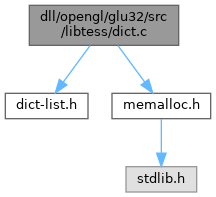dict.c File Reference
`#include "dict-list.h"`
`#include "memalloc.h"`
Include dependency graph for dict.c:Go to the source code of this file.

## Functions

DictdictNewDict (void *frame, int(*leq)(void *frame, DictKey key1, DictKey key2))

void dictDeleteDict (Dict *dict)

DictNodedictInsertBefore (Dict *dict, DictNode *node, DictKey key)

void dictDelete (Dict *dict, DictNode *node)

DictNodedictSearch (Dict *dict, DictKey key)

## ◆ dictDelete()

 void dictDelete ( Dict * dict, DictNode * node )

Definition at line 94 of file dict.c.

95 {
96  node->next->prev = node->prev;
97  node->prev->next = node->next;
98  memFree( node );
99 }
void * next
Definition: dlist.c:360
#define memFree
Definition: memalloc.h:41
Definition: dlist.c:348

## ◆ dictDeleteDict()

 void dictDeleteDict ( Dict * dict )

Definition at line 61 of file dict.c.

62 {
63  DictNode *node, *next;
64
65  for( node = dict->head.next; node != &dict->head; node = next ) {
66  next = node->next;
67  memFree( node );
68  }
69  memFree( dict );
70 }
void * next
Definition: dlist.c:360
struct node node
Definition: dict-list.h:95
#define memFree
Definition: memalloc.h:41
static unsigned __int64 next
Definition: rand_nt.c:6
DictNode * next
Definition: dict-list.h:90
Definition: dlist.c:348

## ◆ dictInsertBefore()

 DictNode* dictInsertBefore ( Dict * dict, DictNode * node, DictKey key )

Definition at line 73 of file dict.c.

74 {
75  DictNode *newNode;
76
77  do {
78  node = node->prev;
79  } while( node->key != NULL && ! (*dict->leq)(dict->frame, node->key, key));
80
81  newNode = (DictNode *) memAlloc( sizeof( DictNode ));
82  if (newNode == NULL) return NULL;
83
84  newNode->key = key;
85  newNode->next = node->next;
86  node->next->prev = newNode;
87  newNode->prev = node;
88  node->next = newNode;
89
90  return newNode;
91 }
DictNode * prev
Definition: dict-list.h:91
void * next
Definition: dlist.c:360
struct node node
HKEY key
Definition: reg.c:42
DictNode * next
Definition: dict-list.h:90
void * frame
Definition: dict-list.h:96
#define NULL
Definition: types.h:112
DictKey key
Definition: dict-list.h:89
int(* leq)(void *frame, DictKey key1, DictKey key2)
Definition: dict-list.h:97
#define memAlloc
Definition: memalloc.h:48
Definition: path.c:41
Definition: dlist.c:348

## ◆ dictNewDict()

 Dict* dictNewDict ( void * frame, int(*)(void *frame, DictKey key1, DictKey key2) leq )

Definition at line 40 of file dict.c.

42 {
43  Dict *dict = (Dict *) memAlloc( sizeof( Dict ));
45
46  if (dict == NULL) return NULL;
47
49
53
54  dict->frame = frame;
55  dict->leq = leq;
56
57  return dict;
58 }
Definition: dict-list.h:95
Definition: dict-list.h:94
void * frame
Definition: dict-list.h:96
#define NULL
Definition: types.h:112
int(* leq)(void *frame, DictKey key1, DictKey key2)
Definition: dict-list.h:97
#define memAlloc
Definition: memalloc.h:48

## ◆ dictSearch()

 DictNode* dictSearch ( Dict * dict, DictKey key )

Definition at line 102 of file dict.c.

103 {
105
106  do {
107  node = node->next;
108  } while( node->key != NULL && ! (*dict->leq)(dict->frame, key, node->key));
109
110  return node;
111 }
void * next
Definition: dlist.c:360
struct node node